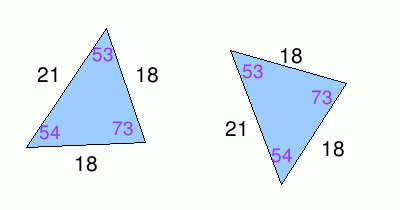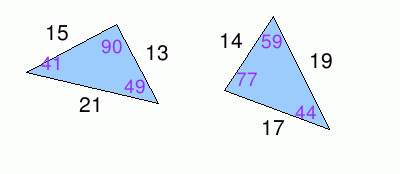MathScore EduFighter is one of the best math games on the Internet today. You can start playing for free!

## Congruent And Similar Triangles - Sample Math Practice Problems

The math problems below can be generated by MathScore.com, a math practice program for schools and individual families. References to complexity and mode refer to the overall difficulty of the problems as they appear in the main program. In the main program, all problems are automatically graded and the difficulty adapts dynamically based on performance. Answers to these sample questions appear at the bottom of the page. This page does not grade your responses.

See some of our other supported math practice problems.

### Complexity=5

Determine whether the following sets of triangles are congruent, similar, or neither.

 1.Congruent        Similar        Neither 2.Congruent        Similar        Neither

### Complexity=5

Determine whether the following sets of triangles are congruent, similar, or neither.

1Congruent
Similar
Neither
Solution
The angle measures are different between the two triangles and so they are neither congruent nor similar.
2